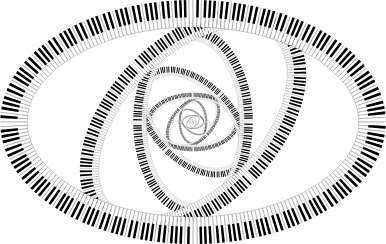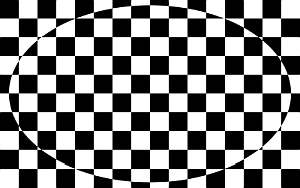# Area of an Ellipse

Instructions: Use this calculator to find the area of an ellipse, with a given semi-axes a and b. Please provide those semi-axes in the boxes below.The semi-major axis $$a$$ of the ellipse (Ex: 2, or 3/2, etc.)The semi-minor axis $$b$$ of the ellipse (Ex: 2, or 3/2, etc.)

This calculator will compute the area of an ellipse, when you provide the semi-major axis and the semi-minor axis of the ellipse. The provided semi-axes of the ellipse must be valid numeric expressions. Then, you can use numbers with or without decimals, such as for example '2' or '4.34', or also you could use valid algebraic expressions like fractions or square roots, such as '2/3', or 'sqrt(7)', etc.

Once you have provided two valid numbers or expressions corresponding to the major and minor semi-axes, then you can click on the "Calculate" button, and you will see all the steps of the process of the calculation of the area.

Knowing how to compute the area of an ellipse and the area of a circle are the basic skills you need to master when dealing with geometric shapes.## How do compute the area of an ellipse?

The formula for the are of the ellipse is uncannily similar to that of the circle, which appears as a remarkable fact. Essentially, all you have to do is multiply "pi" by the product of the semi-axes. The formula is

$\text{Area} = \pi ab$

It is pretty neat how simply it is, huh?

## What are the steps for computing the area of an ellipse

• Step 1: Identify the semi-major axis and the semi-minor axis the ellipse that has been provided, and call them 'a' and 'b'
• Step 2: Once you know the semi-axes 'a' and 'b', the area is computed π a * b
• Step 3: If needed, identify the units of 'a' and 'b' (if any) and give units to the area

## What would be the purpose for the calculation of the area of an ellipse?

Ellipse is a so called conic-form that has a whole lot of applications in real life. Just to give you a little example, the orbit of planets about a star describe an ellipse, so you can imagine that is a quite important application.### Example: Calculate the area of an ellipse

Calculate the area of ellipse with given semi-axes a = 4 and b = 3.

Solution: We need to first identify the major and minor semi-axes sides. In this case, they are a = 4 and b = 3. Then, the formula for the calculation of the area that we need to use is:

$Area = \pi a b$

Then, we plug in the values a = 4 and b = 5 into the formula:

$Area = \pi a b = 4 \times 3 \pi= 12 \pi$

which implies that the area of the ellipse is $$12 \pi$$.

### Example: Area of an with units

Calculate the area of an ellipse with semi-axes given as a = 2.2 cm and b = 3.1 cm

Solution: We first identify the semi-axes. In this case we are given a = 2.2 cm and b = 3.1 cm. So then in this case, we can see that the semi-axes come with length units (cm). The formula is

$Area = \pi a b$

Then, by plugging a = 2.2 cm and b = 3.1 cm into the formula, what we we get is:

$Area = \pi a b = 2.2 \times 3.1 \pi \,\,cm^2 = 6.82 \pi \,\,cm^2$

which indicates that the area that we are looking for is $$6.82 \pi$$ cm2.

## Other practical area calculators

There are geometric shapes that are easier to deal. For example, the calculation of the calculation of the area of the square, the area of the rectangle, the area of a rhombus and the area of a triangle are much easier to compute and to derive, because of fact that their sides are formed by straight lines.

Curved shapes present more challenges. For example, even though the calculation of the area of circle is much more difficult from a conceptual point of view, although the derived formula is remarkably simple, and the same situation occurs for the ellipse.